## Topological Sorting in Java

### Introduction

When getting dressed, as one does, you most likely haven't had this line of thought:

Oh, it might have been a good idea to put on my underpants before getting into my trousers.

That's because we're used to sorting our actions topologically. Or in simpler terms, we're used to logically deducing which actions have to come before or after other actions, or rather which actions are prerequisites for other actions.

For example, let's say that you want to build a house, the steps would look like this:

1. Lay down the foundation
2. Build walls with installations
3. Put in insulation

In that exact order - it's undisputed. You can't build walls if you don't have a foundation, and you can't put in insulation if there aren't any walls.

In this article we will be introducing the idea of Topological Sorting via the following subjects:

### Introduction to Graphs

Since Topological Sorting is applied to Directed Acylic Graphs (DAG), we first have to talk a bit about Graphs.

A Graph is simply a data structure that represents a set of objects that have certain relations between each other - the objects being represented by nodes (circles) and the individual relations by edges (the lines).

There are many different kinds of graphs, but for the problem at hand we need to learn what is a Directed Acyclic Graph. Let's dissect the big bad math term into smaller, more comprehensible segments.

#### Directed

A graph is directed if every relation between 2 objects doesn't have to be bidirectional (it has to have a direction), unlike a unidirectional graph where every relation has to go both ways.

In the graph below, the relation C-A is unidirectional, which means C has a relation with A, and A has a relation with C.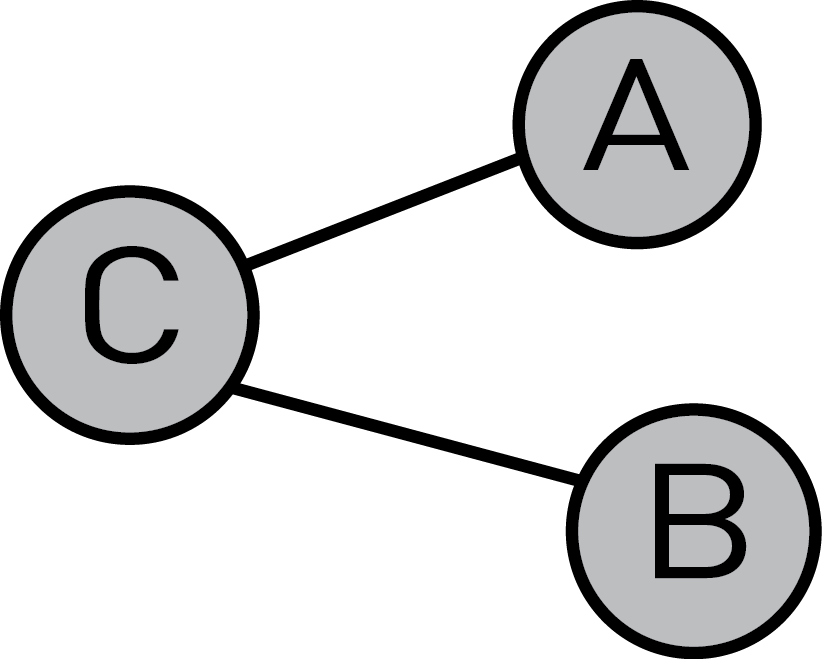On the other hand, in the following graph, the relation C-A is directed, which means A has a relation with C, but C doesn't have a relation with A.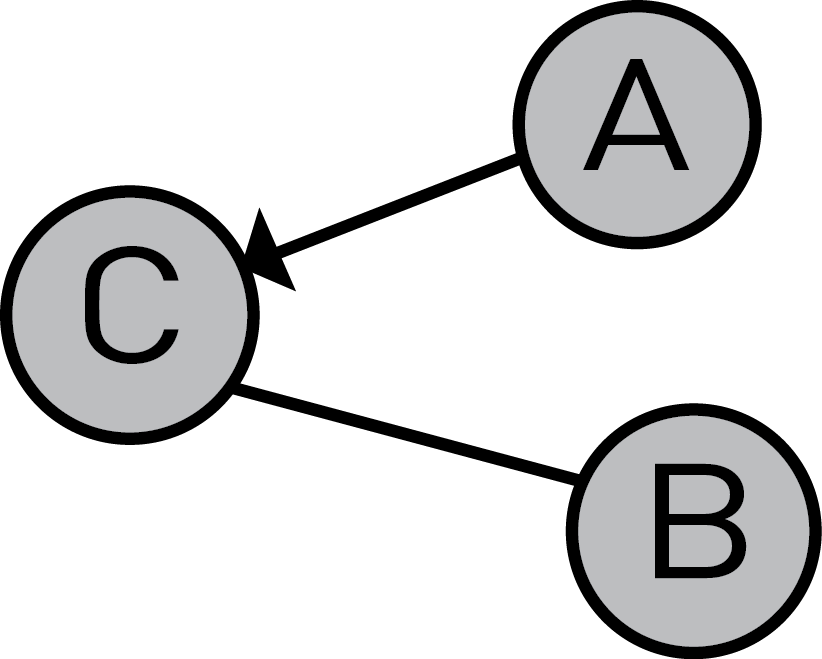Because of this difference, we have to strictly define what are the node's neighbors:

Unidirected graph:

​Two nodes (A and B) are neighboring nodes if a unidirected path exists between them.

Directed graph:

A is B's neighbor if a direct, directed edge exists which leads from B to A. The first direct in this definition refers to the fact that the length of the path leading from B to A has to be strictly 1.

#### Acyclic

A given graph is acyclic only if a cycle does not exist. A cycle is a path for any node X, which starts at X and leads back to X. The following graph is not acyclic because it contains a cycle (X-B-C-X).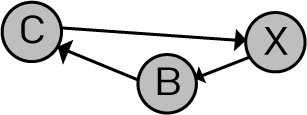### Basic Topological Sorting Concept

So how does topological sorting look when used on a graph, and why does the graph have to be acyclic for it to work?

To answer these questions, let's strictly define what it means to topologically sort a graph:

A graph is topologically sortable if a sequence a1, a2, a3... exists (ai being graph nodes), where for every edge ai->aj, ai comes before aj in the sequence.

If we say that actions are represented by nodes. The above definition would basically mean that an undisputable order of execution must exist.

To better understand the logic behind topological sorting and why it can't work on a graph that contains a cycle, let's pretend we're a computer that's trying to topologically sort the following graph: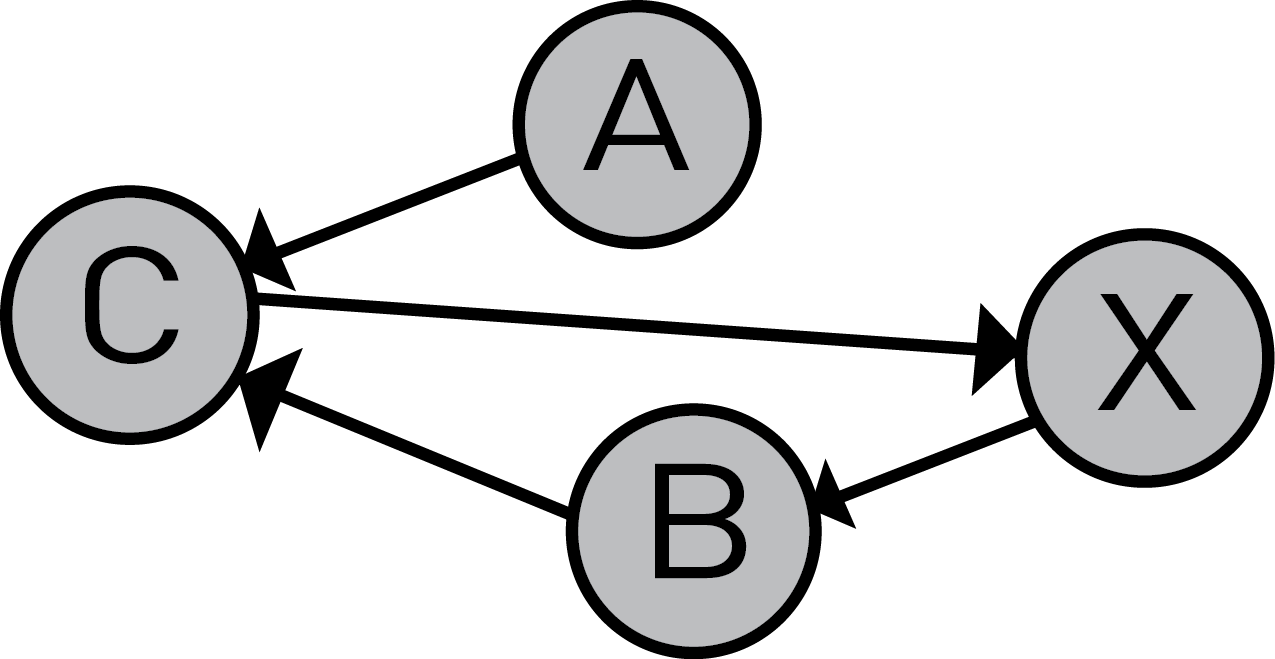# Let's say that we start our search at node X
# Current node: X
step 1: Ok, i'm starting from node X so it must be at the beginnig of the sequence.
sequence: [X]

# The only available edge from X is X->B, so we 'travel' to B
# Current node: B
step 2: Right, B comes after X in the sequence for sure.
sequence: [X,B]

# We travel to C using the edge B->C
# Current node: C
step 3: Same thing as the last step, we add C.
sequence: [X,B,C]

# Current node: X
step 4: WHAT IN THE TARNATION, X AGAIN?
sequence: [X,B,C,X]


This is the reason why we can't topologically sort a graph that contains a cycle, because the following two statements are both true:

• X comes before B
• B comes before X

And because of that, we can't determine an absolute order of the given actions.

Now because we're familiar with the algorithm's concepts, let's take a look at the implementation in Java.

### Implementation

First off, let's construct classes for defining nodes and graphs, and then using said classes, define the following graph: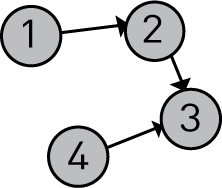public class Graph {
private List<Node> nodes;

public Graph() {
this.nodes = new ArrayList<>();
}

public Graph(List<Node> nodes) {
this.nodes = nodes;
}

}

public List<Node> getNodes() {
return nodes;
}

public Node getNode(int searchId) {
for (Node node:this.getNodes()) {
if (node.getId() == searchId) {
return node;
}
}
return null;
}

public int getSize() {
return this.nodes.size();
}

@Override
public String toString() {
return "Graph{" +
"nodes=" + nodes +
"}";
}
}


The graph is pretty simple, we can instantiate it empty or with a set of nodes, add nodes, retrieve them and print them out.

Now, let's move on to the Node class:

public class Node {
private int id;
private List<Integer> neighbors;

public Node(int id) {
this.id = id;
this.neighbors = new ArrayList<>();
}

}

public int getId() {
return id;
}

public List<Integer> getNeighbors() {
return neighbors;
}

@Override
public String toString() {
return "Node{" +
"id=" + id +
", neighbors=" + neighbors +
"}"+ "\n";
}
}


This class is quite simple as well - just a constructor and a list of neighboring nodes.

With both of our classes, let's instantiate a graph and populate it with a few nodes:

public class GraphInit {
public static void main(String[] args) {
Graph g = new Graph();
Node node1 = new Node(1);
Node node2 = new Node(2);
Node node3 = new Node(3);
Node node4 = new Node(4);
System.out.println(g);
}
}


Output:

Graph{nodes=[Node{id=1, neighbors=}
, Node{id=2, neighbors=}
, Node{id=3, neighbors=[]}
, Node{id=4, neighbors=}
]}


Now let's implement the algorithm itself:

private static void topoSort(Graph g) {

// Fetching the number of nodes in the graph
int V = g.getSize();

// List where we'll be storing the topological order
List<Integer> order = new ArrayList<> ();

// Map which indicates if a node is visited (has been processed by the algorithm)
Map<Integer, Boolean> visited = new HashMap<>();
for (Node tmp: g.getNodes())
visited.put(tmp.getId(), false);

// We go through the nodes using black magic
for (Node tmp: g.getNodes()) {
if (!visited.get(tmp.getId()))
blackMagic(g, tmp.getId(), visited, order);
}

// We reverse the order we constructed to get the
// proper toposorting
Collections.reverse(order);
System.out.println(order);
}

private static void blackMagic(Graph g, int v, Map<Integer, Boolean> visited, List<Integer> order) {
// Mark the current node as visited
visited.replace(v, true);
Integer i;

// We reuse the algorithm on all adjacent nodes to the current node
for (Integer neighborId: g.getNode(v).getNeighbors()) {
if (!visited.get(neighborId))
blackMagic(g, neighborId, visited, order);
}

// Put the current node in the array
}


If we call topoSort(g) for the graph initialized above, we get the following output:

[4, 1, 2, 3]


Which is exactly right.

### Problem Modeling Using Topological Sorting

In a real-world scenario, topological sorting can be utilized to write proper assembly instructions for Lego toys, cars, and buildings.

There's actually a type of topological sorting which is used daily (or hourly) by most developers, albeit implicitly. If you're thinking Makefile or just Program dependencies, you'd be absolutely correct.

A typical Makefile looks like this:

area_51_invasion.out: me.c, the_boys.c, Chads.c, Karen.c, the_manager.c
#instructions for assembly when one of the files in the dependency list is modified


With this line we define which files depend on other files, or rather, we are defining in which topological order the files should be inspected to see if a rebuild is needed.

That is, if area_51_invasion.out depends on the_boys.c and the_boys.c is for some reason modified, we need to rebuild area_51_invasion.out and everything that depends on that same file, that is everything that comes before it in the Makefile's topological order.

### Conclusion

Considering Toposort is basically something we do on a regular basis. You may have even implemented it in your software and didn't even know it. And if you haven't, I strongly suggest you give it a whirl!